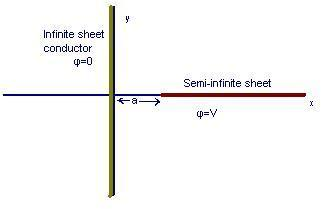# Perpendicular charged sheetsSo, I've solved many problems involving paralell sheets of conductors (finite, semi-infinite, and infinite) and also finite sheets at a given angle to eachother. I can post the results to these if it may be useful, but I'm more interested in sheets that are perpendicular.

Consider a semi-infinite sheet at
$$a\leq x < \infty , y = 0$$
and a perpenciular sheet
$$-\infty < y < \infty , x = 0$$

I'm struggling to find a conformal transformation that will map this problem onto some region (like two infinite paralell lines), from which I can more easily calculate the electrostatic potential and charge density on both sheets.

Any ideas?

#### Attachments

• sheet.bmp
205.8 KB · Views: 388

## Answers and Replies

You ought to use an image charge, also known as the method of images.

How? That works with the symmetry of parallell plates, but there is no such symmetry here.

Can I consider the line of charge as a sum over an infinite number of point charges?